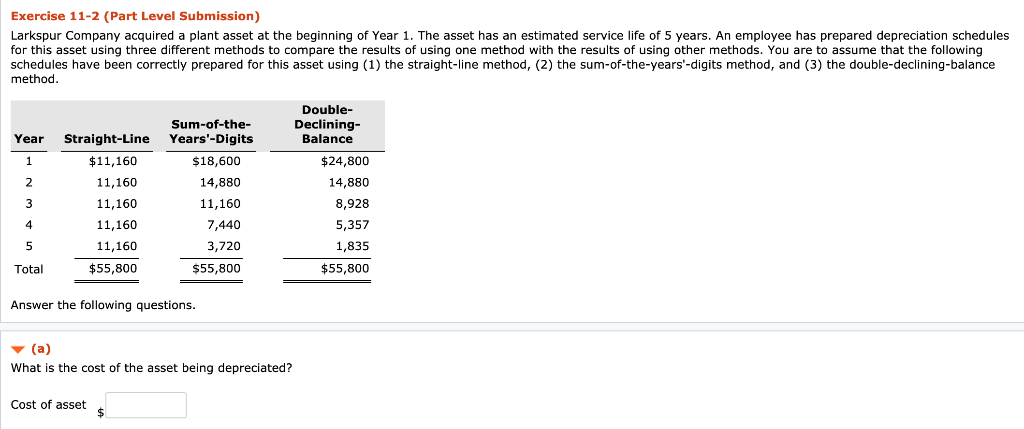### Create an Account

Home / Questions / Answer: Current Ratio Choose Numerator: Choose Denominator: = Current ratio Current Assets...

# Answer: Current Ratio Choose Numerator: Choose Denominator: = Current ratio Current Assets / Current Liabilities = Current ratio Camaro 6000 / 2400 = 2.50 to 1 GTO 3820 / 1410 = 2.71 to 1 Torino 6800Exercise 11-2 (Part Level Submission) Larkspur Company acquired a plant asset at the beginning of Year 1. The asset has an estimated service life of 5 years. An employee has prepared depreciation schedules for this asset using three different methods to compare the results of using one method with the results of using other methods. You are to assume that the following have been correctly prepared for this asset using (1) the straight-line method, (2) the sum-of-the-years&#39;-digits method, and (3) the double-declining-balance method Double- Sum-of-the- Years&#39;-Digits Declining- Balance Year Straight-Line \$18,600 \$11,160 \$24,800 1 2 11,160 14,880 14,880 3 11,160 11,160 8,928 4 11,160 7,440 5,357 3,720 1,835 5 11,160 \$55,800 \$55,800 Total \$55,800 Answer the following questions. (a) What is the cost of the asset being depreciated? Cost of asset

Apr 04 2020 View more View LessSubscribe To Get Solution